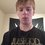# Two Variable Nested Roots Equation

$\sqrt[n]{x \sqrt[n]{ y \sqrt[n]{x \sqrt[n]{y \sqrt[n]{x \sqrt[n]{y \sqrt[n]{x \cdots }}}}}}}$

I noticed something looking at the convergence of geometric series as they relate the questions of the form shown above.

My brain is more empirical and inductive, so I am not as gifted with proofs as the rest of you, nor do I find it easy to tell whether or not something I've found is completely obvious. But what I have done is calculate the convergence of both variables separately. Just using the series test, you can prove convergence, and use any method to determine the actual convergence of a series. I found the series to be in a form: $\frac{1}{x^{n+(n-1)}}$, for example. I simply found a pattern that it seems to stick to, and I believe that just proving that all the series converge is proof enough. Anyway here is the result:

$\sqrt[n]{x \sqrt[n]{ y \sqrt[n]{x \sqrt[n]{y \sqrt[n]{x \sqrt[n]{y \sqrt[n]{x \cdots}}}}}}}= x^{\frac{n}{q}}\cdot y^{\frac{1}{q}}$ Where $\displaystyle q=\sum_{i=2}^{n} n+(n-1)$.

For example: $\sqrt{3\sqrt{2\sqrt{3\sqrt{2 \cdots}}}}=3^{\frac{2}{3}}\cdot 2^{\frac{1}{3}}$

Like I said, I don't know if this is obvious. Somebody probably has already discovered this before, but maybe someone will find it useful.Note by Drex Beckman
5 years, 4 months ago

This discussion board is a place to discuss our Daily Challenges and the math and science related to those challenges. Explanations are more than just a solution — they should explain the steps and thinking strategies that you used to obtain the solution. Comments should further the discussion of math and science.

When posting on Brilliant:

• Use the emojis to react to an explanation, whether you're congratulating a job well done , or just really confused .
• Ask specific questions about the challenge or the steps in somebody's explanation. Well-posed questions can add a lot to the discussion, but posting "I don't understand!" doesn't help anyone.
• Try to contribute something new to the discussion, whether it is an extension, generalization or other idea related to the challenge.
• Stay on topic — we're all here to learn more about math and science, not to hear about your favorite get-rich-quick scheme or current world events.

MarkdownAppears as
*italics* or _italics_ italics
**bold** or __bold__ bold
- bulleted- list
• bulleted
• list
1. numbered2. list
1. numbered
2. list
Note: you must add a full line of space before and after lists for them to show up correctly
paragraph 1paragraph 2

paragraph 1

paragraph 2

[example link](https://brilliant.org)example link
> This is a quote
This is a quote
    # I indented these lines
# 4 spaces, and now they show
# up as a code block.

print "hello world"
# I indented these lines
# 4 spaces, and now they show
# up as a code block.

print "hello world"
MathAppears as
Remember to wrap math in $$ ... $$ or $ ... $ to ensure proper formatting.
2 \times 3 $2 \times 3$
2^{34} $2^{34}$
a_{i-1} $a_{i-1}$
\frac{2}{3} $\frac{2}{3}$
\sqrt{2} $\sqrt{2}$
\sum_{i=1}^3 $\sum_{i=1}^3$
\sin \theta $\sin \theta$
\boxed{123} $\boxed{123}$

## Comments

Sort by:

Top Newest

Good note !!

More or less the same, we can also say that,

\begin{aligned} \sqrt[n]{x \sqrt[n]{ y \sqrt[n]{x \sqrt[n]{y \sqrt[n]{x \sqrt[n]{y \sqrt[n] {x \cdots}}}}}}} & =x^ay^b \\ \Rightarrow \begin{cases} a &=\frac{\frac{1}{n}}{1-\frac{1}{n^2}}=\frac{n}{n^2-1} \\ b & = \frac{\frac{1}{n^2}}{1-\frac{1}{n^2}}=\frac{1}{n^2-1} \end{cases} \end{aligned}

- 5 years, 4 months ago

Log in to reply

Brilliant solution , @Akshat Sharda ! It is very elegant in terms of procedure. It probably makes the calculation a bit easier. I am curious to know your reasoning behind it, if you don't mind. :)

- 5 years, 4 months ago

Log in to reply

×

Problem Loading...

Note Loading...

Set Loading...